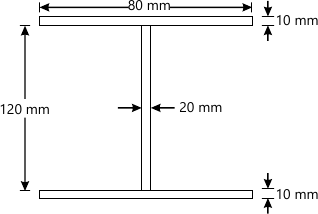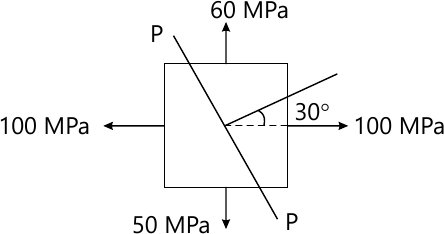MORE IN Strength of Materials
MU Civil Engineering (Semester 3)
Strength of Materials
May 2015
Total marks: --
Total time: --
INSTRUCTIONS
(1) Assume appropriate data and state your reasons
(2) Marks are given to the right of every question
(3) Draw neat diagrams wherever necessary

Attempt any four
1 (a) Derive the relationship between shear force, bending moment and intensity of loading .
5 M
1 (b) If two pieces of material A and B have the same bulk modulus, but the value of modulus of elasticity for B is 1% greater than that for A, find the value of rigidity for the material B in terms of modulus of elasticity and modulus of rigidity for materials A.
5 M
1 (c) Derive an expression for longitudinal stress and circumferential stress for the thin cylinder.
5 M
1 (d) Explain the concept of beam of uniform strength.
5 M
1 (e) State the assumption made in the theory of pure torsion.
5 M

2 (a) A tensile load of 50 kN is acting on the rod of 50mm diameter and length of 5m. Determine the length of a bore of 25mm that can be made central in the rod, if the total extension is not to exceed by 25 percent under the same tensile load. Take E=2.05×105N/mm2
10 M
2 (b) A beam ABCD of span 8m is supported at A,B,and D , has an internal hinge at C. Span AB is of 4m carries a U.D.L. of 20kN/m. Span CD is of 2m carries a U.D.L of 20kN/m find the support reactions and draw the S.F.D and B.M.D
10 M

3 (a) A solid rof of bronze 20 mm in a diameter is surrounded by fitting steel cylinder of external diameter 28mm. If the permissible bending stress in bronze and steel are 100N/mm2 and 150N/mm2, find the moment of resistance of composite section. The Young's modulus for steel may be taken as 1.75 times that of bronze.
10 M
3 (b) What are the principle planes and principle stresses? Explain the Mohr's circle method for two perpendicular direct stresses with state of simple shear.
6 M
3 (c) A beam of triangular cross-section with base b and height h is used with the base horizontal calculate intensity of shear stress at neutral axix and at height h/2. Plot the variation of shear stress intensity over the section.
4 M

4 (a) A circular shaft has to transmit 550kW power at 115RPM . Allowable shear stress=78MPa. Find i) the required diameter of the solid shaft. ii) The dimaeter of the hollow section, such that internal diameter=0.75 external diameter.
10 M
4 (b) A column of 6 m effective length is to be made from 3 plates(fig 8) Using E=210GPa, find the factor of safety with respect to buckling for an axial load of 17kN. Use Euler's theory.6 M
4 (c) Write a note on temperature stresses.
4 M

5 (a) A bar is subjected to a tensile load of 50kN. Bar diameter =32 mm Gauge length=300 mm, extension=0.114mm, change in diameter =0.00366 mm, find poissons ratio, Young's modulus, bulk modulus and modulus of rigidity
6 M
5 (b) Write a short note on fitched beams
4 M
5 (c) An element in a stressed body is subjected to normal stresses on mutually perpendicular directions (fig.9) determine the normal, tangenitial & resultant stresses on a plane P-P inclined as shown. Either use analytical method or graphical method.10 M

6 (a) The steel pipe, 500 mm long is filled with concrete & subjected to an axial compressive load of 90kN. Determine the stress in the concrete and the steel due to this loading. The pipe has on outer diameter=80mm & an inner diameter=70mm E steel =200 Gpa, E concrete=24 Gpa.
6 M
6 (b) Determine the maximum torque that can be applied safely to a solid shaft of diameter 260mm. The permissible angle of twist=1.3 degrees in a length of 6m & the shear stress is not exceed 61 Mpa. Take modulus of rigidity G=90Mpa.
6 M
6 (c) Locate the core or kernel of i-section with the properties top & bottom flange (150mm×10mm), web 280mm × 10mm. Overall depth =300mm.
8 M

More question papers from Strength of Materials